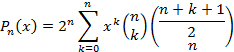Linear Curve Fits

INTRODUCTION

After taking measurements in the presence of noise or other inaccuracies, we often want to find a function which best matches or predicts that data.  In mathematics, the exercise of adjusting parameters in some predicting function for this purpose is called “curve-fitting” or “regression.”

If we draw a straight line through the measured data points, that function is obviously “linear.”  But in a more general sense regression is said to be “linear” if multiplying the parameters by some constant, “c”, increases the total value of the function by that same constant factor. For a single variable “x” and one constant, this meansIn all such cases there is a unique solution for the parameter set.    That is to say for an independent variable “y” that is caused by or “determined” by the dependent variable “x”, we have “n” simultaneous measurements  of both variables  as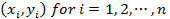We then want to determine the unique set of “m+1” parameters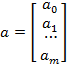that when used in an expansion of a family of functions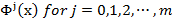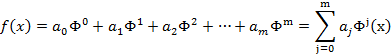will minimize the total squared error between the measurements and functional predictions as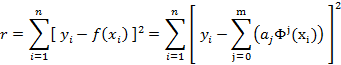The functions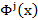are typically a set of orthogonal functions but could simply be a polynomial power series whereorLEAST SQUARES CURVE FIT

We start by taking the derivatives of the squared error with respect to the each one of the “m+1” parameters as followsor by rearranging terms, we have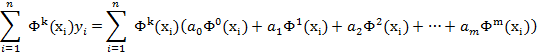These “m+1” equations can then be more concisely expressed in a matrix format as follows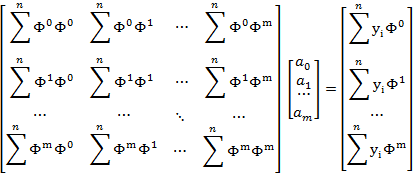which allows for a direct calculation of the parameters using perhaps the Gauss-Jordan method.

LINEAR LEAST-SQUARES FIT

For a linear polynomial or straight line with m=1, then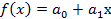and the matrix is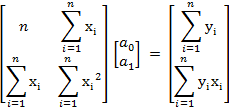This can be directly solved as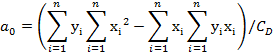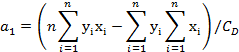For a quadratic polynomial with m=2, then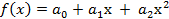and the matrix isBut if we rewrite the matrix as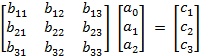then the exact solution is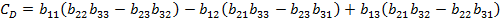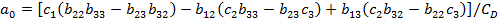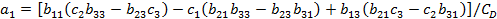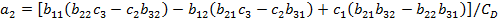LEGENDRE LEAST-SQUARES FIT

The first few Legendre polynomials are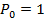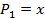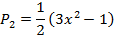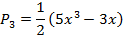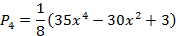And the Legendre polynomials satisfy the recurrence relation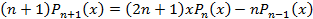or more directly